### Interior Point Algorithm

Primal-dual interior point methods can be classified into two categories: feasible and infeasible. The first category requires that the starting point and all subsequent iterations of the algorithm strictly satisfy all the inequality constraints. The second category relaxes those requirements and allows the iterations to violate some or all of the inequality constraints during the course of the minimization procedure. The NLP solver implements an infeasible algorithm; this section concentrates on that type of algorithm.

To make the notation less cluttered and the fundamentals of interior point methods easier to understand, consider without loss of generality the following simpler NLP problem: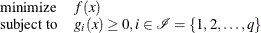Note that the equality and bound constraints have been omitted from the preceding problem. Initially, slack variables are added to the inequality constraints, giving rise to the problem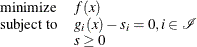where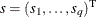is the vector of slack variables, which are required to be nonnegative. Next, all the nonnegativity constraints on the slack variables are eliminated by being incorporated into the objective function, by means of a logarithmic function. This gives rise to the equality-constrained NLP problem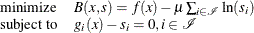whereis a positive parameter. The nonnegativity constraints on the slack variables are implicitly enforced by the logarithmic functions, since the logarithmic function prohibits s from taking zero or negative values.

Next, the equality constraints can be absorbed by using a quadratic penalty function to obtain the following: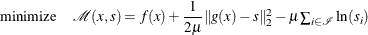The preceding unconstrained problem is often called the penalty-barrier subproblem. Depending on the size of the parameter, a local minimum of the barrier problem provides an approximation to the local minimum of the original NLP problem. The smaller the size of, the better the approximation becomes. Infeasible primal-dual interior point algorithms repeatedly solve the penalty-barrier problem for different values ofthat progressively go to zero, in order to get as close as possible to a local minimum of the original NLP problem.

An unconstrained minimizer of the penalty-barrier problem must satisfy the equations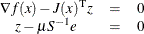where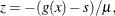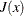is the Jacobian matrix of the vector function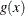, S is the diagonal matrix whose elements are the elements of the vector s (that is,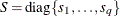), and e is a vector of all ones. Multiplying the second equation by S and adding the definition of z as a third equation produces the following equivalent nonlinear system: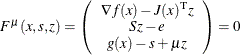Note that if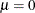, the preceding conditions represent the optimality conditions of the original optimization problem, after adding slack variables. One of the main aims of the algorithm is to gradually reduce the value ofto zero, so that it converges to a local optimum of the original NLP problem. The rate by whichapproaches zero affects the overall efficiency of the algorithm. Algorithms that treat z as an additional variable are considered primal-dual, while those that enforce the definition of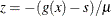at each iteration are consider purely primal approaches.

At iteration k, the infeasible primal-dual interior point algorithm approximately solves the preceding system by using Newton’s method. The Newton system is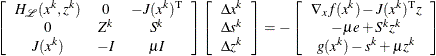where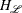is the Hessian matrix of the Lagrangian function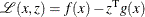of the original NLP problem; that is,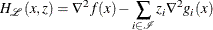The solution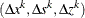of the Newton system provides a direction to move from the current iteration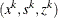to the next,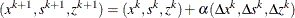where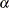is the step length along the Newton direction. The step length is determined through a line-search procedure that ensures sufficient decrease of a merit function based on the augmented Lagrangian function of the barrier problem. The role of the merit function and the line-search procedure is to ensure that the objective and the infeasibility reduce sufficiently at every iteration and that the iterations approach a local minimum of the original NLP problem.